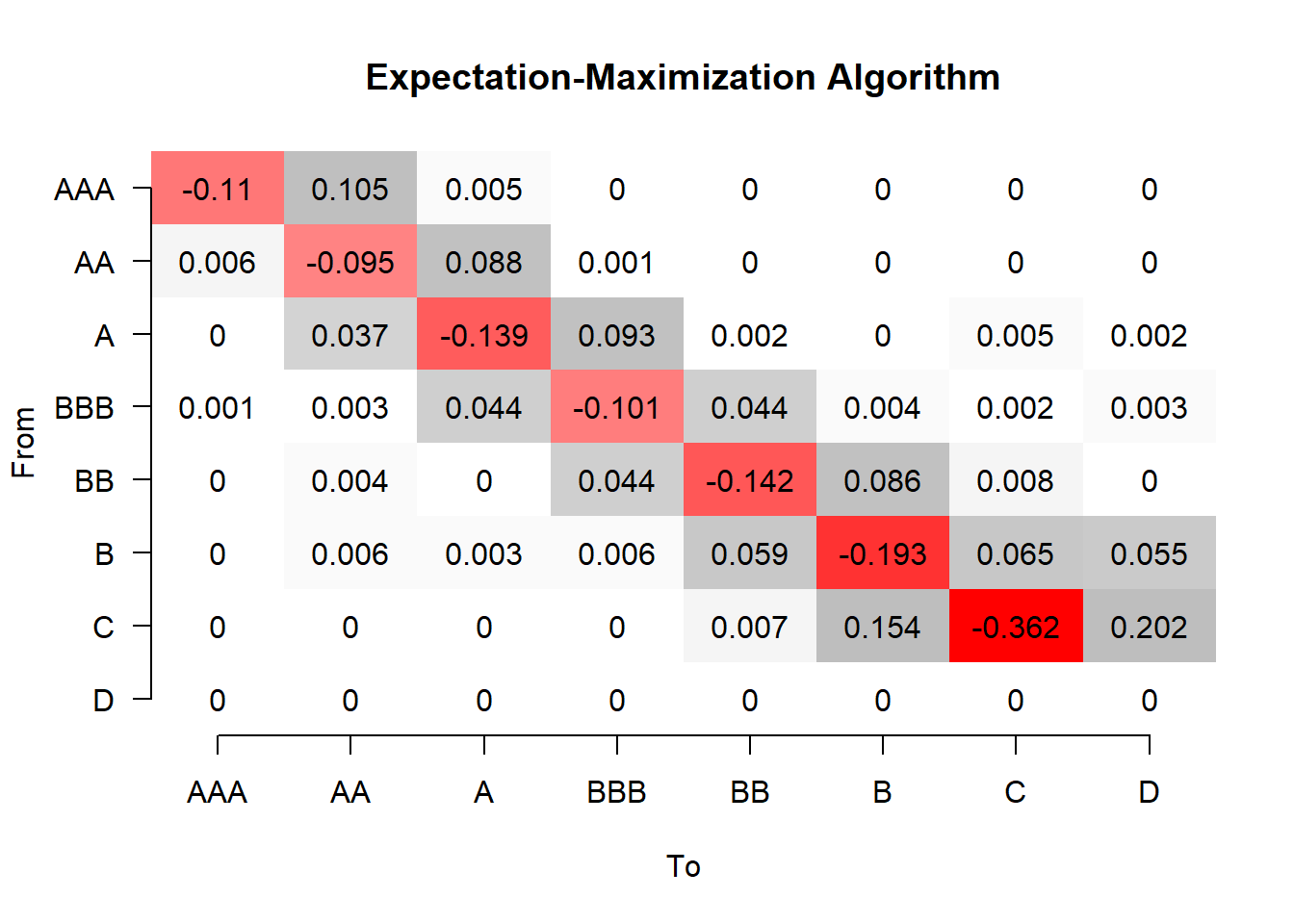A ctmcd Guide

Introduction

ctmcd is a package for the estimation of Markov generator matrices (i.e., the parameters of a continuous-time Markov chain) for the special case, that only discrete-time observations are given. The implemented methods to derive these estimates are diagonal adjustment (“DA”, Israel et al., 2001), weighted adjustment (“WA”, Israel et al., 2001), quasi-optimization (“QO”, Kreinin and Sidelnikova, 2001), an instance of the expectation-maximization algorithm (“EM”, Bladt and Soerensen, 2005) and a Gibbs Sampler (“GS”, Bladt and Soerensen, 2005). For the expectation-maximization algorithm a Wald confidence interval according to the method of Oakes, 1999 can be derived. For the posterior mean estimate from the Gibbs sampler, an equal tailed credibility interval as outlined in Bladt and Soerensen, 2009 is implemented.

library(ctmcd)

Generator Matrix Estimation

In order to perform a generator matrix estimate, the discrete time data must be available as a matrix of either absolute (“EM”, “GS”) or relative transition frequencies (“DA”, “WA”, “QO”), depending on the method employed.

data(tm_abs)

loads a credit transition example data set. In order to perform a diagonal adjustment generator matrix estimate, this data has to be converted into a matrix of relative transition frequencies first. Then, the gm method can be employed, requiring the time elapsed in the discrete time transition process, which is 1 as the example data has a single-year time horizon and furthermore a method specification, which is “DA” in this case.

tm_rel=rbind((tm_abs/rowSums(tm_abs))[1:7,],c(rep(0,7),1))
gmda=gm(tm=tm_rel,te=1,method="DA")
gmda
## Call:
## gm.default(tm = tm_rel, te = 1, method = "DA")
##
## Parameters:
##            AAA        AA         A       BBB         BB          B
## AAA -1.100e-01  0.104900  0.005093  0.000000  4.585e-06  5.828e-07
## AA   6.495e-03 -0.095770  0.088150  0.001133  0.000e+00  0.000e+00
## A    0.000e+00  0.037630 -0.139300  0.092890  2.105e-03  3.269e-05
## BBB  6.568e-04  0.003008  0.043670 -0.101100  4.438e-02  4.164e-03
## BB   0.000e+00  0.004096  0.000000  0.044050 -1.428e-01  8.617e-02
## B    0.000e+00  0.005848  0.003293  0.005807  5.893e-02 -1.932e-01
## C    2.429e-06  0.000000  0.000000  0.000000  7.001e-03  1.551e-01
##      0.000e+00  0.000000  0.000000  0.000000  0.000e+00  0.000e+00
##             C        D
## AAA  0.000000 0.000000
## AA   0.000000 0.000000
## A    0.004585 0.002025
## BBB  0.001778 0.003400
## BB   0.008452 0.000000
## B    0.064440 0.054920
## C   -0.363400 0.201300
##      0.000000 0.000000

A maximum likelihood estimate can be obtained by the EM algorithm, additionally requiring a starting value gmguess.

gm0=matrix(1,8,8)
diag(gm0)=0
diag(gm0)=-rowSums(gm0)
gm0[8,]=0

gmem=gm(tm_abs,te=1,method="EM",gmguess=gm0)
gmem
## Call:
## gm.default(tm = tm_abs, te = 1, method = "EM", gmguess = gm0)
##
## Parameters:
##            AAA         AA          A        BBB         BB          B
## AAA -1.095e-01  1.049e-01  4.613e-03  5.717e-22  5.984e-32  2.089e-30
## AA   6.231e-03 -9.500e-02  8.785e-02  9.160e-04  7.664e-22  1.258e-24
## A    3.698e-17  3.749e-02 -1.389e-01  9.294e-02  2.000e-03  4.502e-06
## BBB  6.155e-04  3.016e-03  4.358e-02 -1.010e-01  4.439e-02  4.173e-03
## BB   1.187e-16  4.050e-03  9.687e-06  4.387e-02 -1.424e-01  8.606e-02
## B    1.986e-18  5.769e-03  3.231e-03  5.734e-03  5.894e-02 -1.930e-01
## C    9.053e-21  5.933e-08  1.652e-08  2.460e-08  6.780e-03  1.541e-01
## D    0.000e+00  0.000e+00  0.000e+00  0.000e+00  0.000e+00  0.000e+00
##              C         D
## AAA  4.326e-31 5.286e-39
## AA   2.367e-21 1.333e-27
## A    4.501e-03 1.961e-03
## BBB  1.779e-03 3.400e-03
## BB   8.403e-03 1.912e-09
## B    6.452e-02 5.476e-02
## C   -3.624e-01 2.015e-01
## D    0.000e+00 0.000e+00

Confidence / Credibility Intervals

Interval estimates of gm objects can only be obtained for the methods “EM” and “GS” by means of the gmci function, e.g., a 95% Wald confidence interval can be obtained by

ciem=gmci(gmem,alpha=0.05)

Matrix Plot Function

Both, gm and gmci objects can be visualized by the matrix plot function plotM(), which can be easily accessed by the wrapper function plot().

plot(gmem)